# RD Sharma Solutions for Class 10 Chapter 1 Real Numbers Exercise 1.5

Irrational numbers are the numbers which cannot be expressed in the standard form of p/q. Proving the irrationality of numbers is clearly explained in this exercise. Our subjects experts at BYJU’S have created the RD Sharma Solutions Class 10 to make students understand the correct procedure to solve the exercise problems. In case you need any reference to any question of this exercise, you can access the RD Sharma Solutions for Class 10 Maths Chapter 1 Real Numbers Exercise 1.5 for which PDF is available below.

## RD Sharma Solutions for Class 10 Chapter 1 Real Numbers Exercise 1.5 Download PDF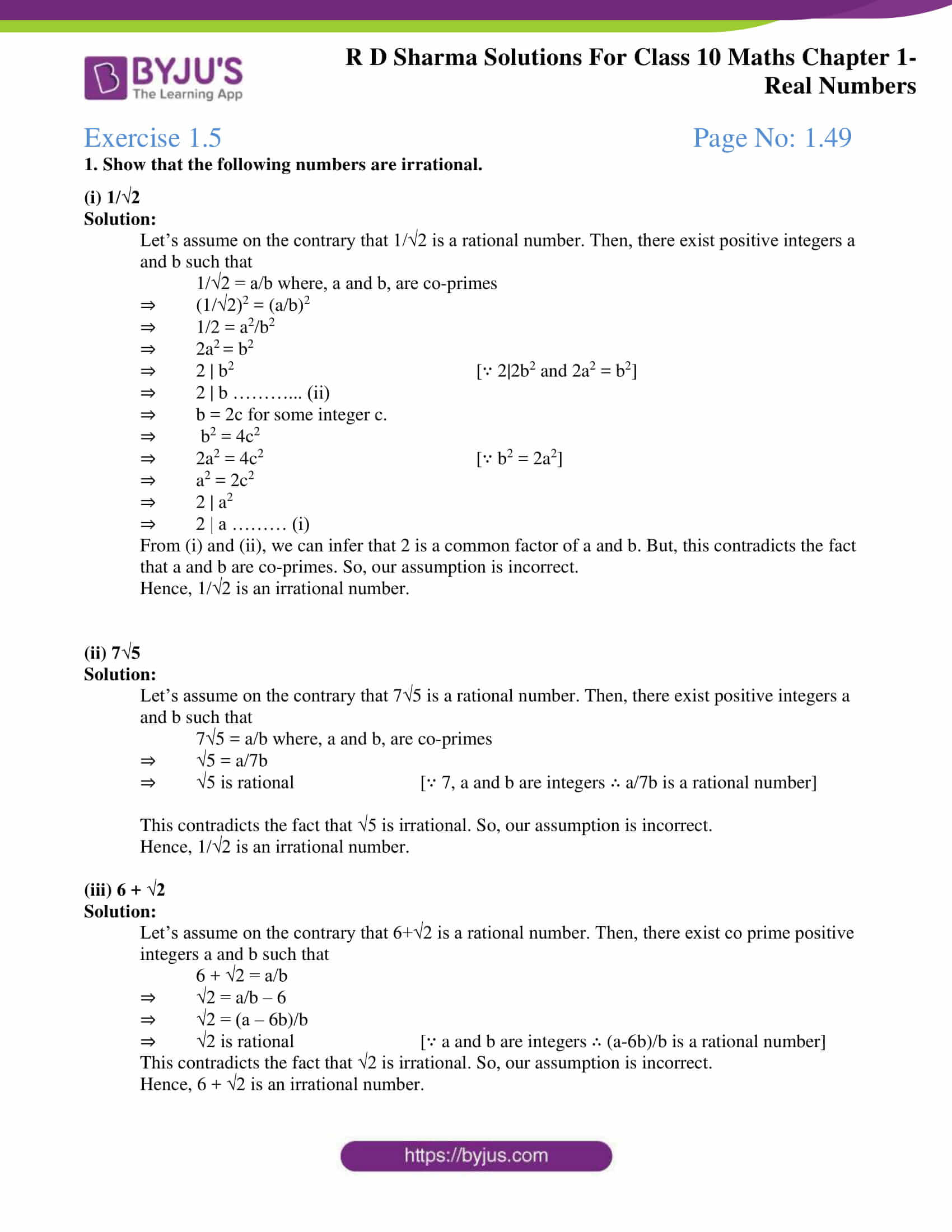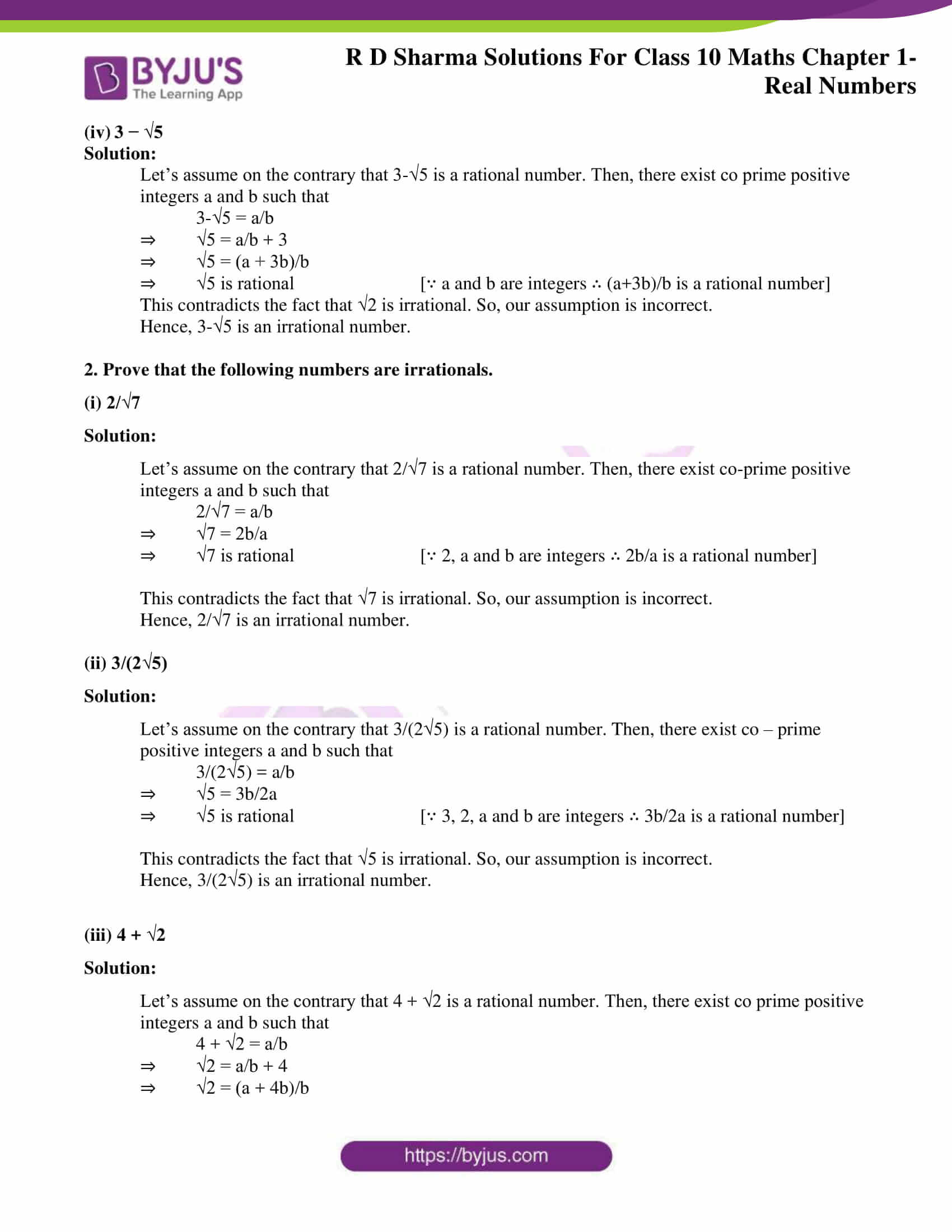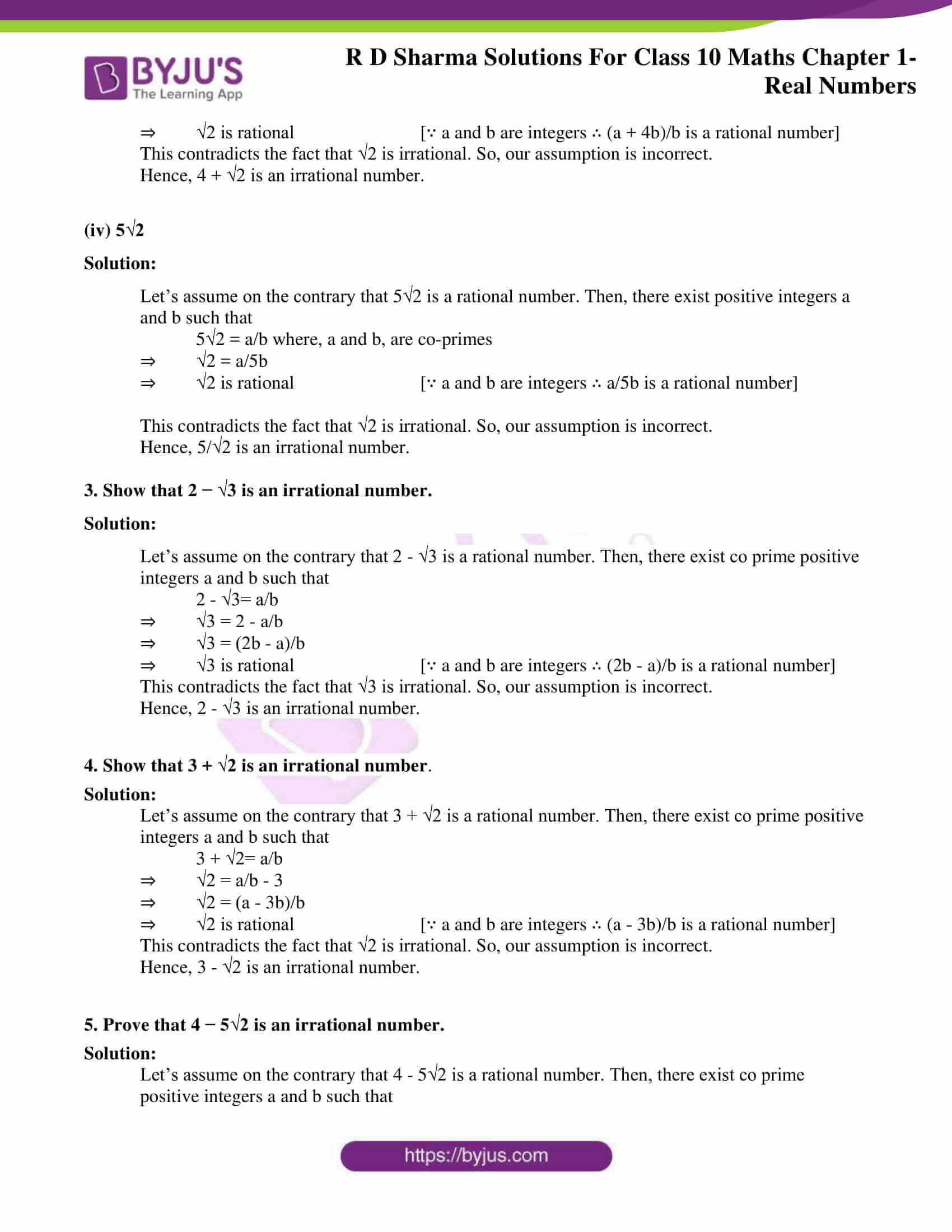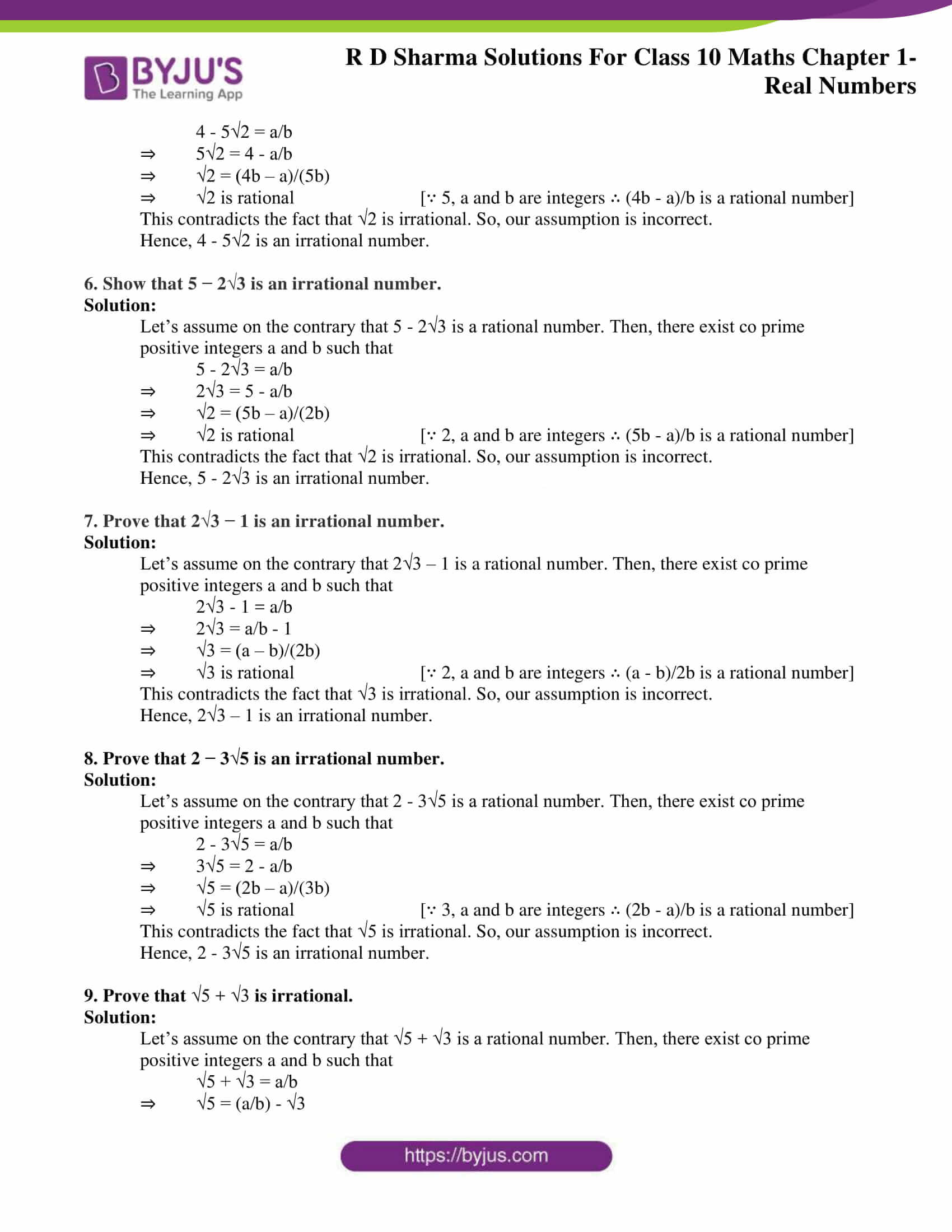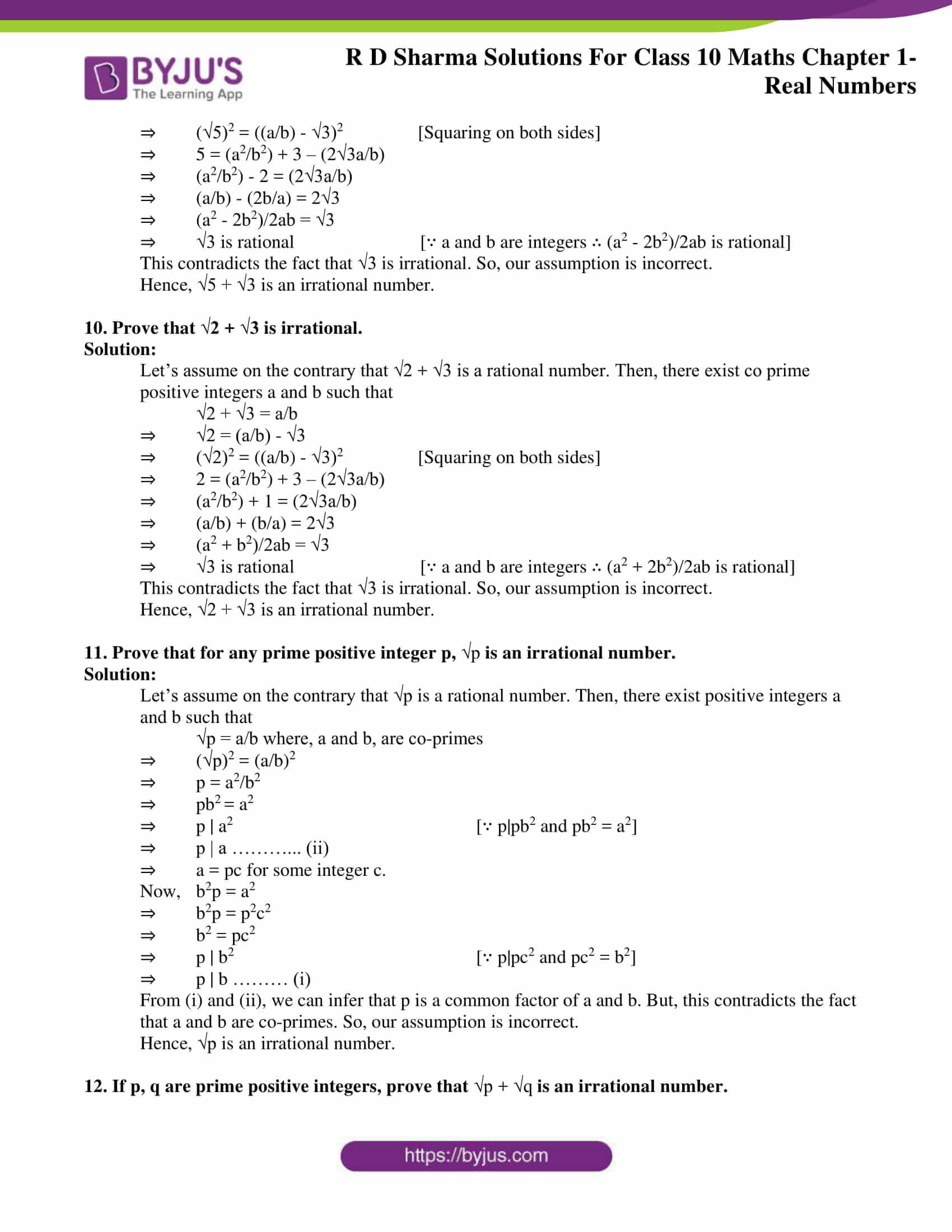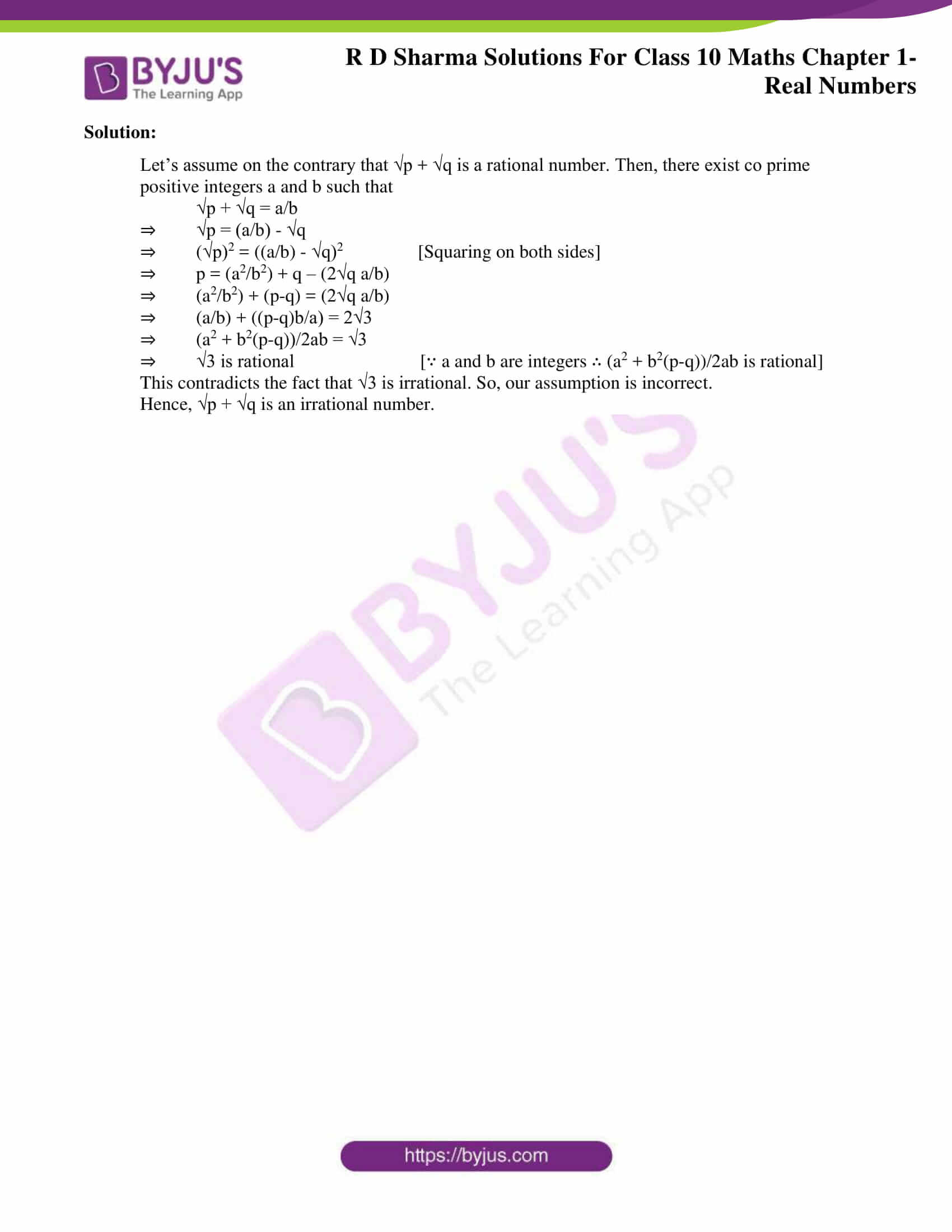### Access RD Sharma Solutions for Class 10 Chapter 1 Real Numbers Exercise 1.5

1. Show that the following numbers are irrational.

(i) 1/√2

Solution:

Let’s assume on the contrary that 1/√2 is a rational number. Then, there exist positive integers a and b such that

1/√2 = a/b where, a and b, are co-primes

⇒ (1/√2)2 = (a/b)2

⇒ 1/2 = a2/b2

⇒ 2a2 = b2

⇒ 2 | b2 [∵ 2|2b2 and 2a2 = b2]

⇒ 2 | b ………… (ii)

⇒ b = 2c for some integer c.

⇒ b2 = 4c2

⇒ 2a2 = 4c2 [∵ b2 = 2a2]

⇒ a2 = 2c2

⇒ 2 | a2

⇒ 2 | a ……… (i)

From (i) and (ii), we can infer that 2 is a common factor of a and b. But, this contradicts the fact that a and b are co-primes. So, our assumption is incorrect.

Hence, 1/√2 is an irrational number.

(ii) 7√5

Solution:

Let’s assume on the contrary that 7√5 is a rational number. Then, there exist positive integers a and b such that

7√5 = a/b where, a and b, are co-primes

⇒ √5 = a/7b

⇒ √5 is rational [∵ 7, a and b are integers ∴ a/7b is a rational number]

This contradicts the fact that √5 is irrational. So, our assumption is incorrect.

Hence, 1/√2 is an irrational number.

(iii) 6 + √2

Solution:

Let’s assume on the contrary that 6+√2 is a rational number. Then, there exist co prime positive integers a and b such that

6 + √2 = a/b

⇒ √2 = a/b – 6

⇒ √2 = (a – 6b)/b

⇒ √2 is rational [∵ a and b are integers ∴ (a-6b)/b is a rational number]

This contradicts the fact that √2 is irrational. So, our assumption is incorrect.

Hence, 6 + √2 is an irrational number.

(iv) 3 − √5

Solution:

Let’s assume on the contrary that 3-√5 is a rational number. Then, there exist co prime positive integers a and b such that

3-√5 = a/b

⇒ √5 = a/b + 3

⇒ √5 = (a + 3b)/b

⇒ √5 is rational [∵ a and b are integers ∴ (a+3b)/b is a rational number]

This contradicts the fact that √2 is irrational. So, our assumption is incorrect.

Hence, 3-√5 is an irrational number.

2. Prove that the following numbers are irrationals.

(i) 2/√7

Solution:

Let’s assume on the contrary that 2/√7 is a rational number. Then, there exist co-prime positive integers a and b such that

2/√7 = a/b

⇒ √7 = 2b/a

⇒ √7 is rational [∵ 2, a and b are integers ∴ 2b/a is a rational number]

This contradicts the fact that √7 is irrational. So, our assumption is incorrect.

Hence, 2/√7 is an irrational number.

(ii) 3/(2√5)

Solution:

Let’s assume on the contrary that 3/(2√5) is a rational number. Then, there exist co – prime positive integers a and b such that

3/(2√5) = a/b

⇒ √5 = 3b/2a

⇒ √5 is rational [∵ 3, 2, a and b are integers ∴ 3b/2a is a rational number]

This contradicts the fact that √5 is irrational. So, our assumption is incorrect.

Hence, 3/(2√5) is an irrational number.

(iii) 4 + √2

Solution:

Let’s assume on the contrary that 4 + √2 is a rational number. Then, there exist co prime positive integers a and b such that

4 + √2 = a/b

⇒ √2 = a/b + 4

⇒ √2 = (a + 4b)/b

⇒ √2 is rational [∵ a and b are integers ∴ (a + 4b)/b is a rational number]

This contradicts the fact that √2 is irrational. So, our assumption is incorrect.

Hence, 4 + √2 is an irrational number.

(iv) 5√2

Solution:

Let’s assume on the contrary that 5√2 is a rational number. Then, there exist positive integers a and b such that

5√2 = a/b where, a and b, are co-primes

⇒ √2 = a/5b

⇒ √2 is rational [∵ a and b are integers ∴ a/5b is a rational number]

This contradicts the fact that √2 is irrational. So, our assumption is incorrect.

Hence, 5/√2 is an irrational number.

3. Show that 2 − √3 is an irrational number.

Solution:

Let’s assume on the contrary that 2 – √3 is a rational number. Then, there exist co prime positive integers a and b such that

2 – √3= a/b

⇒ √3 = 2 – a/b

⇒ √3 = (2b – a)/b

⇒ √3 is rational [∵ a and b are integers ∴ (2b – a)/b is a rational number]

This contradicts the fact that √3 is irrational. So, our assumption is incorrect.

Hence, 2 – √3 is an irrational number.

4. Show that 3 + √2 is an irrational number.

Solution:

Let’s assume on the contrary that 3 + √2 is a rational number. Then, there exist co prime positive integers a and b such that

3 + √2= a/b

⇒ √2 = a/b – 3

⇒ √2 = (a – 3b)/b

⇒ √2 is rational [∵ a and b are integers ∴ (a – 3b)/b is a rational number]

This contradicts the fact that √2 is irrational. So, our assumption is incorrect.

Hence, 3 – √2 is an irrational number.

5. Prove that 4 − 5√2 is an irrational number.

Solution:

Let’s assume on the contrary that 4 – 5√2 is a rational number. Then, there exist co prime positive integers a and b such that

4 – 5√2 = a/b

⇒ 5√2 = 4 – a/b

⇒ √2 = (4b – a)/(5b)

⇒ √2 is rational [∵ 5, a and b are integers ∴ (4b – a)/b is a rational number]

This contradicts the fact that √2 is irrational. So, our assumption is incorrect.

Hence, 4 – 5√2 is an irrational number.

6. Show that 5 − 2√3 is an irrational number.

Solution:

Let’s assume on the contrary that 5 – 2√3 is a rational number. Then, there exist co prime positive integers a and b such that

5 – 2√3 = a/b

⇒ 2√3 = 5 – a/b

⇒ √2 = (5b – a)/(2b)

⇒ √2 is rational [∵ 2, a and b are integers ∴ (5b – a)/b is a rational number]

This contradicts the fact that √2 is irrational. So, our assumption is incorrect.

Hence, 5 – 2√3 is an irrational number.

7. Prove that 2√3 − 1 is an irrational number.

Solution:

Let’s assume on the contrary that 2√3 – 1 is a rational number. Then, there exist co prime positive integers a and b such that

2√3 – 1 = a/b

⇒ 2√3 = a/b – 1

⇒ √3 = (a – b)/(2b)

⇒ √3 is rational [∵ 2, a and b are integers ∴ (a – b)/2b is a rational number]

This contradicts the fact that √3 is irrational. So, our assumption is incorrect.

Hence, 2√3 – 1 is an irrational number.

8. Prove that 2 − 3√5 is an irrational number.

Solution:

Let’s assume on the contrary that 2 – 3√5 is a rational number. Then, there exist co prime positive integers a and b such that

2 – 3√5 = a/b

⇒ 3√5 = 2 – a/b

⇒ √5 = (2b – a)/(3b)

⇒ √5 is rational [∵ 3, a and b are integers ∴ (2b – a)/b is a rational number]

This contradicts the fact that √5 is irrational. So, our assumption is incorrect.

Hence, 2 – 3√5 is an irrational number.

9. Prove that √5 + √3 is irrational.

Solution:

Let’s assume on the contrary that √5 + √3 is a rational number. Then, there exist co prime positive integers a and b such that

√5 + √3 = a/b

⇒ √5 = (a/b) – √3

⇒ (√5)2 = ((a/b) – √3)2 [Squaring on both sides]

⇒ 5 = (a2/b2) + 3 – (2√3a/b)

⇒ (a2/b2) – 2 = (2√3a/b)

⇒ (a/b) – (2b/a) = 2√3

⇒ (a2 – 2b2)/2ab = √3

⇒ √3 is rational [∵ a and b are integers ∴ (a2 – 2b2)/2ab is rational]

This contradicts the fact that √3 is irrational. So, our assumption is incorrect.

Hence, √5 + √3 is an irrational number.

10. Prove that √2 + √3 is irrational.

Solution:

Let’s assume on the contrary that √2 + √3 is a rational number. Then, there exist co prime positive integers a and b such that

√2 + √3 = a/b

⇒ √2 = (a/b) – √3

⇒ (√2)2 = ((a/b) – √3)2 [Squaring on both sides]

⇒ 2 = (a2/b2) + 3 – (2√3a/b)

⇒ (a2/b2) + 1 = (2√3a/b)

⇒ (a/b) + (b/a) = 2√3

⇒ (a2 + b2)/2ab = √3

⇒ √3 is rational [∵ a and b are integers ∴ (a2 + 2b2)/2ab is rational]

This contradicts the fact that √3 is irrational. So, our assumption is incorrect.

Hence, √2 + √3 is an irrational number.

11. Prove that for any prime positive integer p, √p is an irrational number.

Solution:

Let’s assume on the contrary that √p is a rational number. Then, there exist positive integers a and b such that

√p = a/b where, a and b, are co-primes

⇒ (√p)2 = (a/b)2

⇒ p = a2/b2

⇒ pb2 = a2

⇒ p | a2 [∵ p|pb2 and pb2 = a2]

⇒ p | a ………… (ii)

⇒ a = pc for some integer c.

Now, b2p = a2

⇒ b2p = p2c2

⇒ b2 = pc2

⇒ p | b2 [∵ p|pc2 and pc2 = b2]

⇒ p | b ……… (i)

From (i) and (ii), we can infer that p is a common factor of a and b. But, this contradicts the fact that a and b are co-primes. So, our assumption is incorrect.

Hence, √p is an irrational number.

12. If p, q are prime positive integers, prove that √p + √q is an irrational number.

Solution:

Let’s assume on the contrary that √p + √q is a rational number. Then, there exist co prime positive integers a and b such that

√p + √q = a/b

⇒ √p = (a/b) – √q

⇒ (√p)2 = ((a/b) – √q)2 [Squaring on both sides]

⇒ p = (a2/b2) + q – (2√q a/b)

⇒ (a2/b2) + (p-q) = (2√q a/b)

⇒ (a/b) + ((p-q)b/a) = 2√3

⇒ (a2 + b2(p-q))/2ab = √3

⇒ √3 is rational [∵ a and b are integers ∴ (a2 + b2(p-q))/2ab is rational]

This contradicts the fact that √3 is irrational. So, our assumption is incorrect.

Hence, √p + √q is an irrational number.